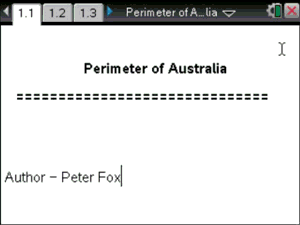# Activities

•• ##### Subject Area

• Standard: National Curriculum 7-10: Number and Algebra: Patterns and algebra
• Standard: National Curriculum 7-10: Number and Algebra: Linear and non-Linear Relationships

• ##### AuthorMiddle

90 Minutes

• ##### Device
•TI-Nspire™ CX
•TI-Nspire™ CX CAS
• ##### Software

TI-Nspire™
TI-Nspire™ CAS

3.2

• ##### Report an Issue

Perimeter of Australia#### Activity Overview

Students plot points on a map of Australia drawn on the Cartesian plane. The coordinates of the points are used to determine the distance between successive points and the progressive distance (perimeter) of Australia.

#### Objectives

• ACMNA214 – Find the distance between two points located on a Cartesian plane using a range of strategies, including graphing software.
• Elaborations: Investigate graphically and algebraically, techniques for finding distance between two points using Pythagoras' theorem.

#### Vocabulary

• Pythagoras
• Distance between two points
• Cartesian plane
• Coordinate geometry

#### About the Lesson

Students plot points on the graph, TI-nspire determines the coordinates. The formula for the distance between two points is written on the screen. Students connect the appropriate coordinates to the formula and the answer is computed by TI-nspire. In their written notes students do the same providing for both technology support and independent determination of the distance between two points.